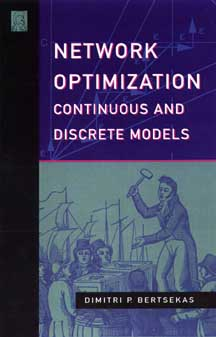# Network Optimization: Continuous and Discrete Models

ISBN: 1-886529-02-7
Publication: MAY 1998, 608 pages, hardcover
Price: \$69.00

Network Optimization Codes from the author's websiteAn insightful, comprehensive, and up-to-date treatment of linear, nonlinear, and discrete/combinatorial network optimization problems, their applications, and their analytical and algorithmic methodology.

It covers extensively theory, algorithms, and applications, and it aims to bridge the gap between linear and nonlinear network optimization on one hand, and integer/combinatorial network optimization on the other. It complements several of our books: Convex Optimization Theory (Athena Scientific, 2009), Convex Optimization Algorithms (Athena Scientific, 2015), Introduction to Linear Optimization (Athena Scientific, 1997), Nonlinear Programming (Athena Scientific, 1999), as well as our other book on the subject of network optimization Network Flows and Monotropic Optimization (Athena Scientific, 1998).

From the review by Panos Pardalos (Optimization Methods and Sofware, 2000):

"This beautifully written book provides an introductory treatment of linear, nonlinear, and discrete network optimization problems... The textbook is addressed not only to students of optimization but to all scientists in numerous disciplines who need network optimization methods to model and solve problems. This book is an engaging read and it is highly recommended either as a textbook or as a reference on network optimization."

Among its special features, the book:

• provides a comprehensive account of the principal algorithms for linear network flow problems, including simplex, dual ascent, and auction algorithms

• describes the application of network algorithms in many practical contexts, with special emphasis on data communication networks

• develops in detail the computational complexity analysis of the main linear network optimization algorithms

• covers extensively the main algorithms for specialized network problems, such as shortest path, max-flow, assignment, and traveling salesman

• describes the main models for discrete network optimization problems, such as constrained shortest path, traveling salesman, vehicle routing, multidimensional assignment, facility location, spanning tree construction, etc

• describes the main algorithmic approaches for integer-constrained network problems, such as branch-and-bound, Lagrangian relaxation and subgradient optimization, genetic algorithms, tabu search, simulated annealing, and rollout algorithms

• develops the main methods for nonlinear network problems, such as convex separable and multicommodity flow problems arising in communication, transportation, and manufacturing contexts

• discusses extensively auction algorithms, based on the author's original research on the subject

• contains many examples, practical applications, illustrations, and exercises